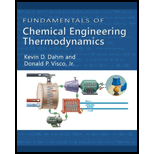Chapter 4.8, Problem 10P### Fundamentals of Chemical Engineeri...

1st Edition
Kevin D. Dahm + 1 other
ISBN: 9781111580704

#### Solutions

Chapter
Section### Fundamentals of Chemical Engineeri...

1st Edition
Kevin D. Dahm + 1 other
ISBN: 9781111580704
Textbook Problem

# A series of two turbines and a heat exchanger are used to obtain shaft work from steam in a steady-state process. The steam enters the first turbine at 10 bar and 250°C, and exits the turbine at 3 bar. In the heat exchanger, the steam is heated back up to 250°C, while the pressure remains 3 bar. This steam enters the second turbine in which it is expanded to 1 bar. Each turbine has an efficiency of 80%. For each kilogram of steam entering the process, find the amount of work produced in each turbine, and the amount of heat added in the heat exchanger.

Interpretation Introduction

Interpretation:

Determine the work produced in first and second turbine and the amount of heat added to the heat exchanger.

Concept Introduction:

The expression for an entropy balance equation.

d(MS^)dt=j=1j=Jm˙j,inS^jk=1k=Km˙k,outS^k+n=1n=NQ˙nTn+S˙gen

Here, time taken is t, mass of the system is M, specific entropy of the system are S^, mass flow rates of individual streams entering and leaving the system are m˙j,in and m˙k,out, specific entropies of streams entering and leaving the system are S^j and S^k, actual rate at which heat is added to or removed from the system at one particular location is Q˙n, the temperature of the system at the boundary where the heat transfer labelled n occurs is Tn, and the rate at which entropy is generated within the boundaries of the system is S˙gen.

Write an energy balance for an adiabatic steady state turbine.

W˙Sm˙=H^outH^in

Here, specific enthalpy at inlet and outlet are H^in and H^out, mass flow rate is m˙, and rate at which shaft work is added to the system is W˙S.

Write the efficiency of the turbine.

η=W˙S,actualm˙W˙S,revm˙

Here, actual work done by the shaft is W˙S,actual and reversible work done by the shaft is W˙S,rev.

Explanation

Given information:

First turbine pressure and temperature is 10 bar and 250 degree Celsius.

Second turbine pressure and temperature is 3 bar and 250 degree Celsius with efficiency of 80%.

Refer the Appendix table A-3, “Superheated steam”, obtain the value of inlet properties of specific enthalpy and specific entropy at a pressure of 10 bar and temperature of 250°C respectively.

H^in=2943.1kJ/kgS^in=6.9265kJ/kgK

Calculate the inlet entropy using entropy balance equation.

d(MS^)dt=j=1j=Jm˙j,inS^jk=1k=Km˙k,outS^k+n=1n=NQ˙nTn+S˙gen        (1)

Rewrite the steady state entropy balance equation for an adiabatic ideal mass system 0=m˙inS^inm˙outS^out+S˙gen0=S^inS^out+0S^in=S^out        (2)

Substitute 6.9265kJ/kgK for S^in in Equation (2).

6.9265kJ/kgK=S^outS^out=6.9265kJ/kgK

Refer the Appendix table A-1, “Saturated steam-pressure increments”, obtain the specific entropy and specific enthalpy for liquid and vapor state at a pressure of 3 bar.

S^L=1.6717kJ/kgKS^V=6.9916kJ/kgKH^L=561.4kJ/kgH^V=2724.9kJ/kg

Calculate the specific entropy of an outlet for a reversible process.

S^out,rev=(1qrev)S^L+qrevS^V        (3)

Here, mole fraction of the system for reversible process is qrev.

Substitute 1.6717kJ/kgK for S^L, 6.9916kJ/kgK for S^V, and 6.9265kJ/kgK for S^out in Equation (3).

6.9265kJ/kgK=(1qrev)1.6717kJ/kgK+qrev(6.9916kJ/kgK)qrev=0.988

Calculate the enthalpy for outlet steam in reversible process.

H^out,rev=(1qrev)H^L+qrevH^V        (4)

Substitute 561.4kJ/kg for H^L, 2724.9kJ/kg for H^V, and 0.988 for qrev in Equation (4)

H^out,rev=(10.988)561.4kJ/kg+0.988(2724.9kJ/kg)=2698.4kJ/kg

Calculate the work done for an adiabatic steady state turbine.

W˙Sm˙=H^outH^in        (5)

Substitute 2698.4kJ/kg for H^out,rev and 2724.9kJ/kg for H^in in Equation (5).

W˙S,revm˙=H^out,revH^in=2,698.4kJ/kg2724

### Still sussing out bartleby?

Check out a sample textbook solution.

See a sample solution

#### The Solution to Your Study Problems

Bartleby provides explanations to thousands of textbook problems written by our experts, many with advanced degrees!

Get Started

## Additional Engineering Solutions

#### Find more solutions based on key concepts